# Place Value: Decimals

Decimals are a shorthand way to write fractions and mixed numbers with denominators that are powers of $10$ , like $10,100,1000,10000,$ etc.

If a number has a decimal point , then the first digit to the right of the decimal point indicates the number of tenths.

For example, the decimal $0.3$ is the same as the fraction $\frac{3}{10}$ .

The second digit to the right of the decimal point indicates the number of hundredths.

For example, the decimal $3.26$ is the same as the mixed number $3\frac{26}{100}$ . (Note that the first digit to the left of the decimal point is the ones digit.)

You can write decimals with many places to the right of the decimal point. For example, this is a representation of the mixed number $51\frac{48053}{1000000}$ , with the place values named: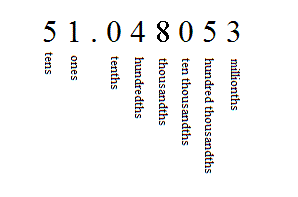One good way to visualize decimals is by using base $10$ blocks. For instance, suppose a large square respresents one whole. If the square is cut into $10$ strips of equal size, then each of these represents one tenth or $0.1$ . Each strip can be cut into ten smaller squares to represent hundredths.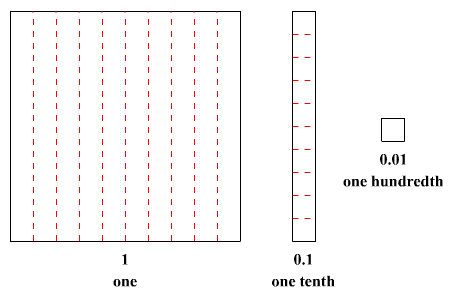Example 1:

What number does the following set of blocks represent?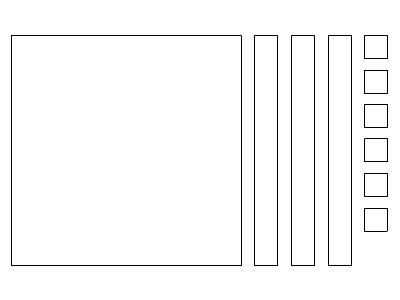There is $1$ large square, so the ones digit is $1$ . There are $3$ one-tenths strips, so the tenths digit is $3$ . There are $6$ one-hundredth squares, so the hundredths digit is $6$ .

So, the figure represents the decimal $1.36$ .

Example 2:

What number does the following diagram represent?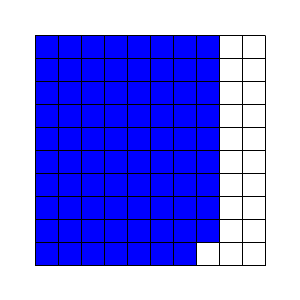The large square is divided into $100$ squares. Seven complete rows have been shaded (that is, seven tenths) along with nine squares of another row (nine hundredths). So, the decimal number is $0.79$ .

Example 3:

If the large square represents one whole, what decimal number does the diagram represent?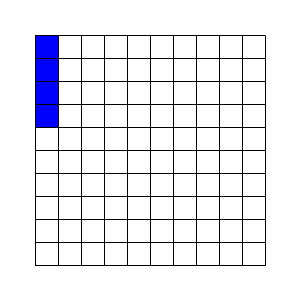The large square is divided into $100$ squares. No complete rows have been shaded (that is, zero tenths) but four small squares of one row have been shaded (four hundredths). So, the decimal number is $0.04$ .